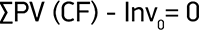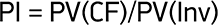investment
calculators

## NPV calculator (Net Present Value of project)

### Calculator

%
{{ calcNVP.dataSetCount() }} year
Period IC CI CO CF DCF
0
{{ \$index + 1 }}
NPV

NPV > 0, project should be accepted NPV < 0, project should be cancelled

### Calculation method

The net present value of the investment project is immediate change in the value of company when project is accepted.ICO - Initial investment

CH n - Cash flow of n-year

NPV - Net present valueMethod rule: If NPV >= 0 – project should be accepted, if NPV < 0 – project should be cancelled. When NPV is positive, the project creates value, when it's negative – destroy.

## DPP calculator (Discount payback period)

### Calculator

%
{{ calcDPP.dataSetCount() }} year
Period IC CI CO CF DCF
0
{{ \$index + 1 }}

DPP (years): {{ calcDPP.calcDPPValue() | number:2 }}. Payback period is longer than expected period, it's better to reject it

### Calculation method

Payback period - is the period when cash flow exceeds invested capital.DPP - Period when the condition will be sutisfied:## PI calculator (Profitability index of project)

### Calculator

%
{{ calcPI.dataSetCount() }} year
Period IC CI CO CF DCF
0
{{ \$index + 1 }}

PI = {{ calcPI.calcPI() | number:2 }}, capital investment is effective, the project can be accepted PI = {{ calcPI.calcPI() | number:2 }}, capital investment is not effective, it's better to reject the project

### Calculation method

The profitability index is an index that attempts to identify the relationship between the costs and benefits of a proposed project.